# Consolidating Lists While Adding Links to the Source Data in Microsoft Excel

If you have data in the same cells in multiple sheets that need to be summed up together, you can do this using a very simple technique.  And if you have a range of cells that you need to sum, you can sum one cell and then drag the formula down or right or copy it to those ranges, which need to be summed.

Let’s see how this technique works

Considering that we have a workbook that has 3 sheets – January, February, and March.

There are values in cells A2: A4 in each of these sheets.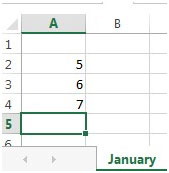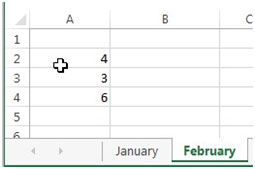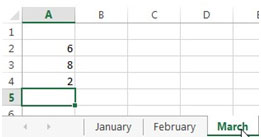Now in another sheet, we need to sum up the values in each of the cells. So we need to sum A2 in January, February, and March.  Respectively for A3 and A4 in this example.

So in a blank sheet, type =SUM and then click on the January tab.  Hold the shift key on the keyboard and select the 3rd sheet – March.  Select the cell A2 and press Enter on your keyboard.

The formula will now show =SUM(January: March!A2)

You will get the sum of the cell A2 from January to March. Now drag down this formula to A4 and the values will be populated.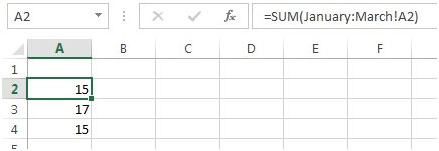In this example, we had values only in A2: A4.  However, if you have values horizontally, in columns B onwards, you can drag the formula right. Or copy the formula to other cells where you need to sum up the values from the corresponding sheets.

There is another method to sum the values from cells in different sheets. We can use the 3D reference option.

3D Reference:  This option is used to combine the data from the different sheets in a single sheet. 3D reference contains three dimensions - rows, columns, and worksheets.

Let’s take an example, we have sales data for 3 months in 3 different sheets in an Excel workbook, and in one sheet, we will return the total quantity of all months agent wise.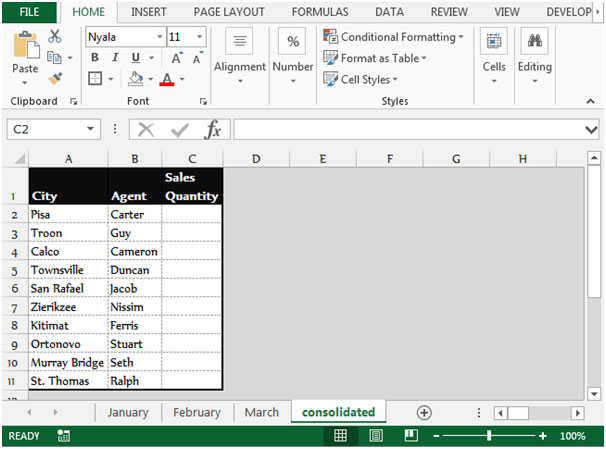Follow the below given steps to sum the values from the cells in different sheets -

• Select the cell C2 in the “consolidated” sheet
• Go to the Data tab, and select Consolidate from the Data tools group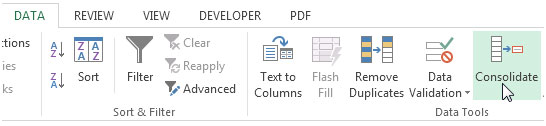• The Consolidate dialog box will appear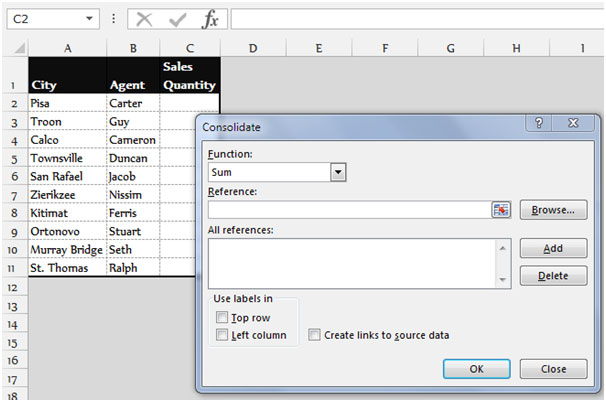• Select Sum from the function drop down list
• Click on Reference, select the January sheet and then select the range C2:C11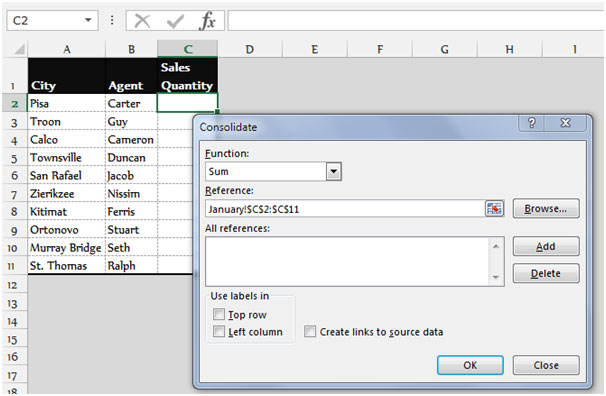• Click on add button and then select the range C2:C11 in the February sheet
• Click on add button and then select the range C2:C11 in the March sheet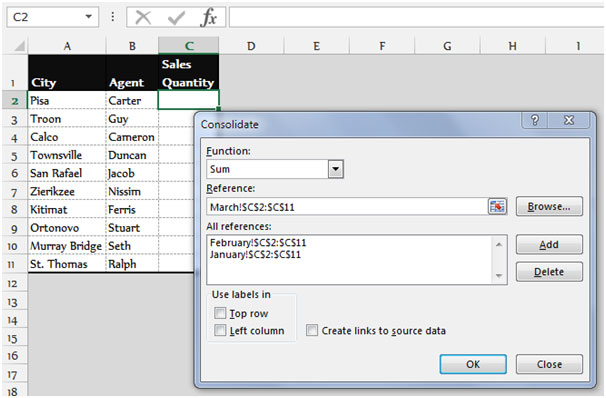• Click on OK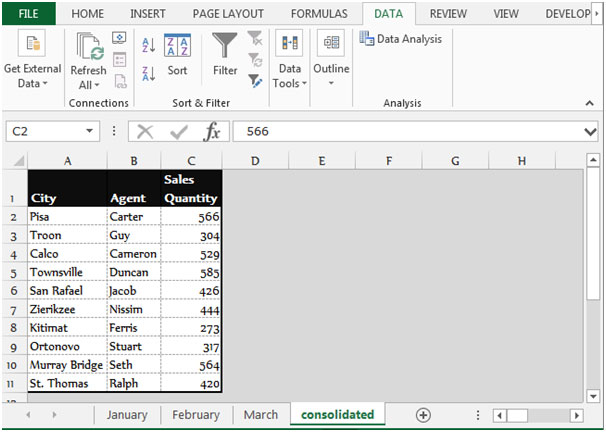• The total sales quantity will appear in the range C2:C11 for all the agents